# Developing the basics: Programming myself, post fourteen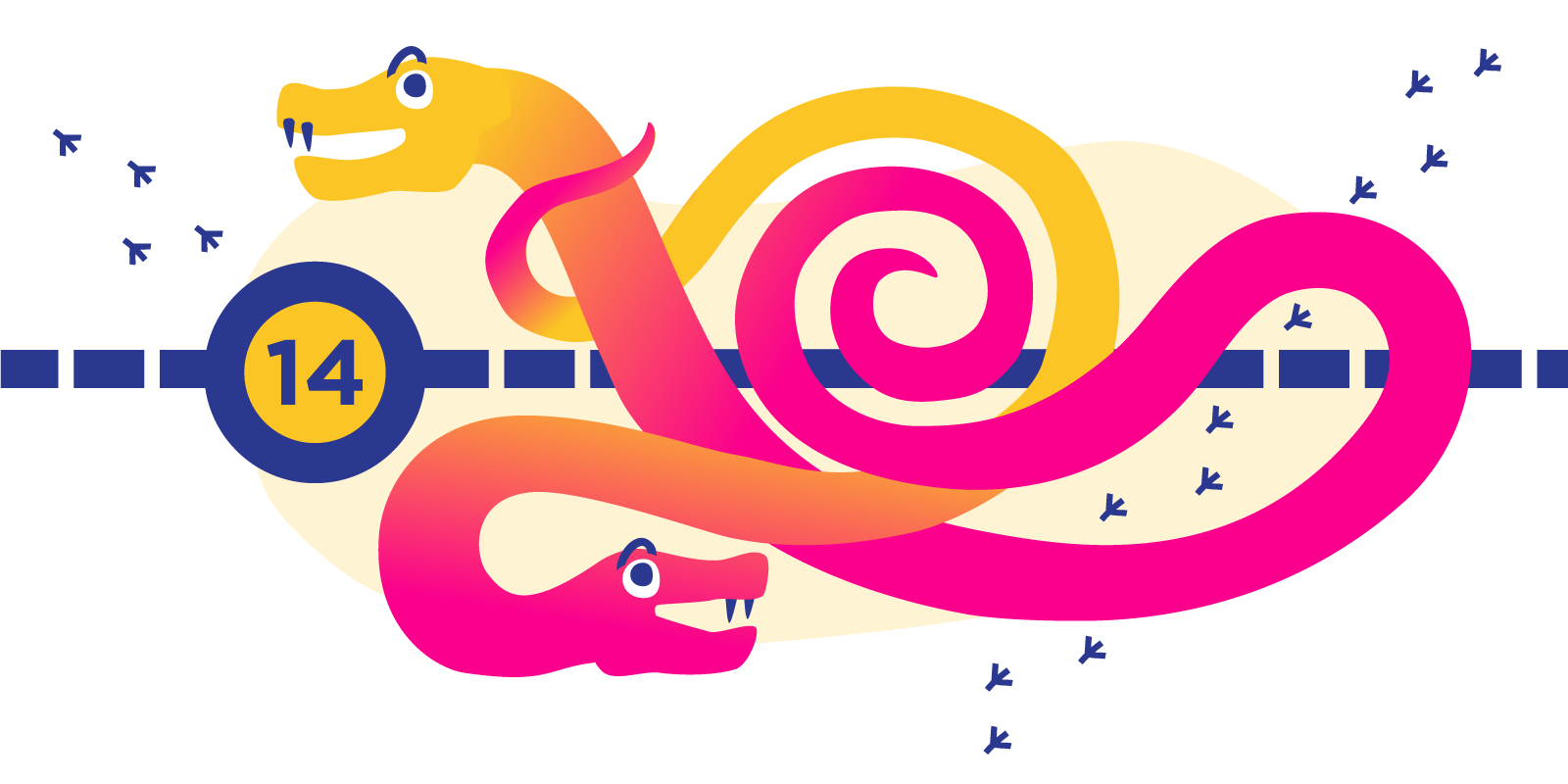Now comes the really fun part, putting all the things that have been learned over the past 5 months into practice. It should be a nice final finish to the process of learning how to program as we can see what we know and test out what we have learned, but also see where there are still gaps in our knowledge which we can work on in the future. My final challenge then is to take the Adaptive Python Course which runs with PyCharm Edu and the online learning platform Stepik and finish up my quest on learning to program to the level I set out to achieve. The platform has a few different courses available for different programming languages like Kotlin and Java, but we are getting right back to what we came here for, Python.

So the first question I guess you have is:

This course basically doesn’t have a set way of getting through it. You progress through the exercises depending on how you did with the previous exercises. The main idea behind this is that you are challenged, not to the point where you give up in frustration and throw your computer out of the window, but just enough to keep you interested at your own personal level. This is great, as normally exercises can be kind of boring in a static format as they have to assume your level of understanding already and so take you through things that maybe don’t interest you enough as they cannot change (adapt) to your level, so it offers you a way to progress at you own bespoke pace. Stepik description. The course itself uses coding practice which includes some theory you get tasks and hints, and you just have to go through and put something together which works for the problem at hand… 428 times…
So first things first, you need to log into Stepik and enroll for the course.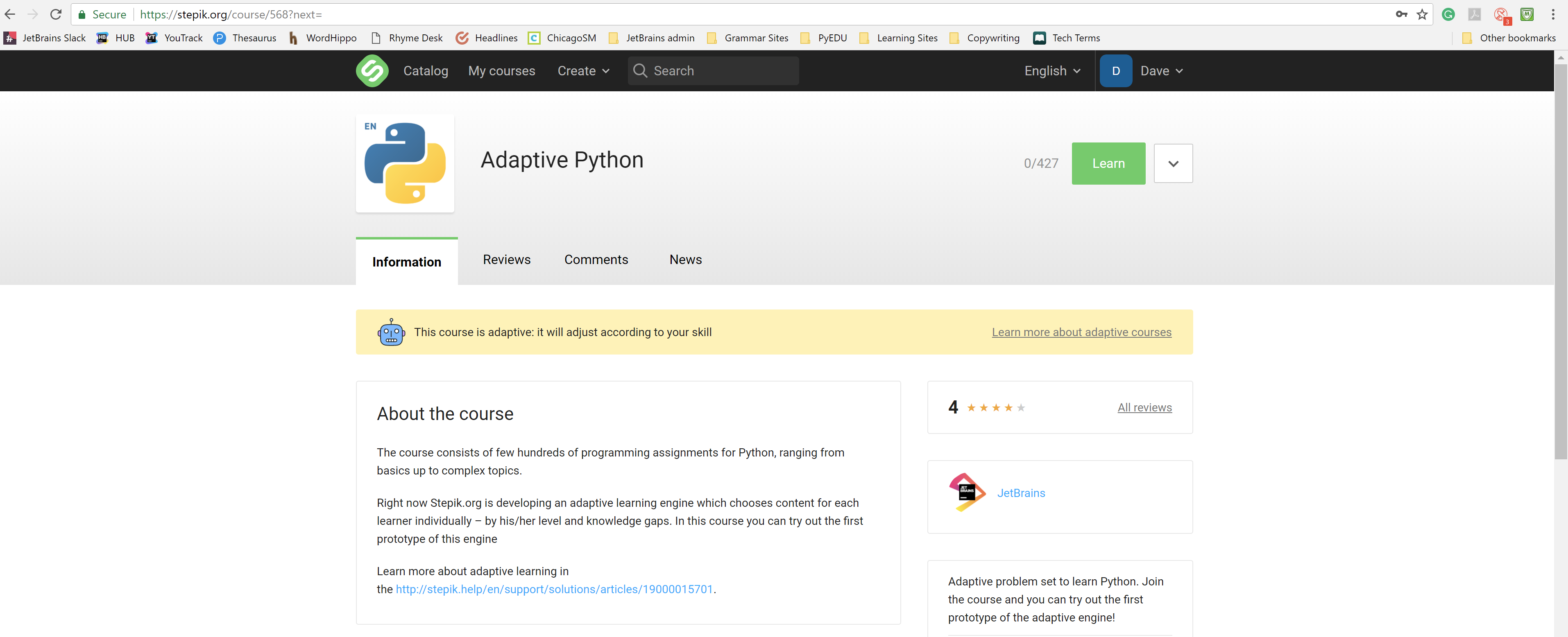## Do it in PyCharm Edu

Actually, I don’t really want to do this course on the Stepik platform itself but in the PyCharm Edu, so easy enough open up the PyCharm Edu, File… Browse courses Adaptive Python – Join.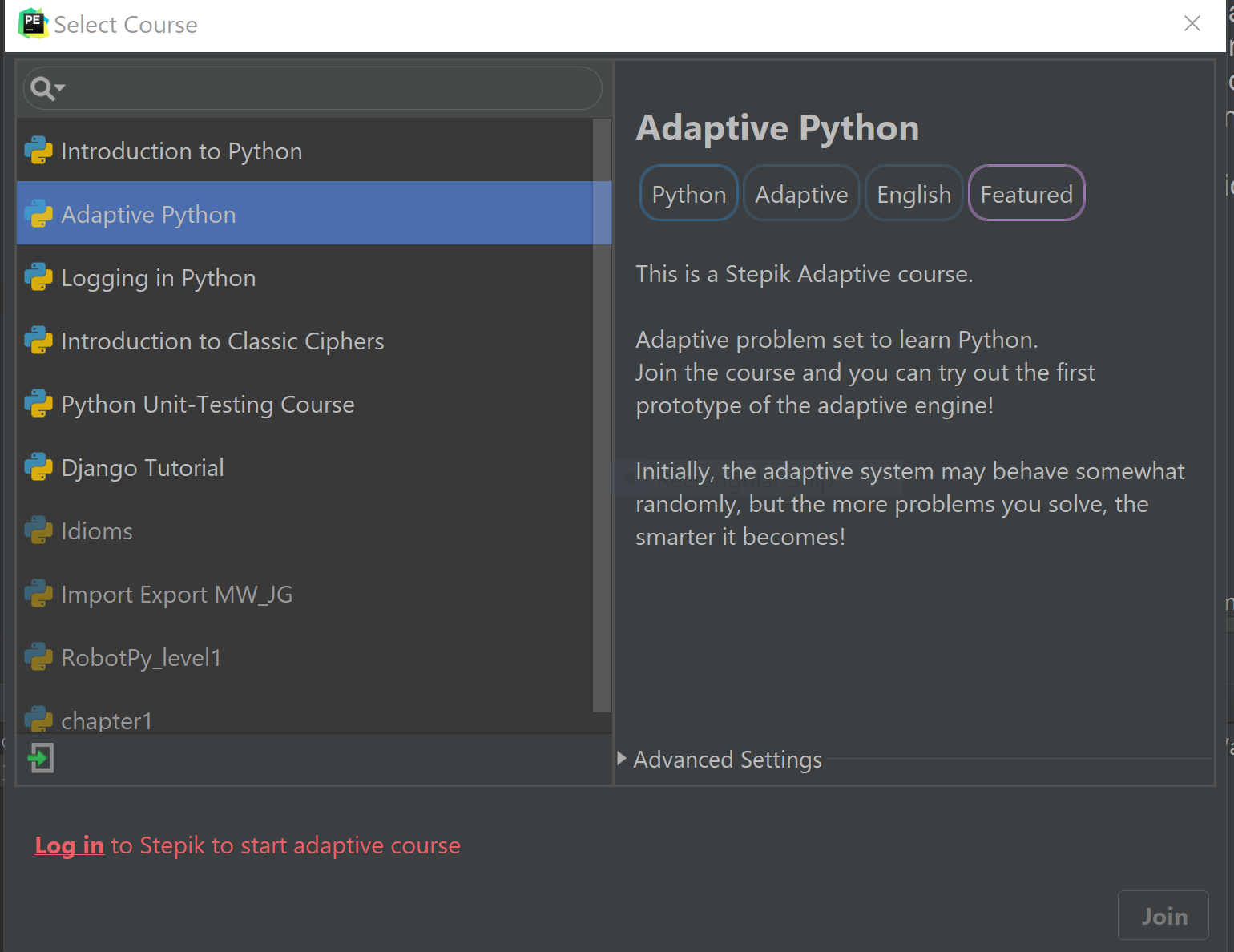## Don’t get scared now

I immediately regret this decision, and the cold realization hits me that this is going to be harder than just following through examples in a book. “You need to generate all possible k-combinations of n elements” for the first question.

As we talked about the other week the problem solving is now starting to kick in, it is uncomfortable, it is frustrating, and because of this it is worth doing and will obviously (hopefully) pay off. This really is something though that needs to be done to break away from the plateau of passive learning if you feel you are not really gaining enough to be able to consider yourself able to program.

This really is completely different from all the courses I have completed so far as it is total problem solving from scratch. There are no hints to begin with, and it is really crippling to realize how even though I think I have a good grasp of Python and the theory of computer science, this is the actual practice that is needed, and that there is still a lot to learn.

I guess the best place to start is declaring the variables k and n and their relation to one another… 0 < k < n.
We need to use input to input the numbers, and we need to somehow consider all the sequences of numbers up to n…

We need to print out as many lines as the variable k. So I guess then a “for loop” will be needed in there somewhere.

So as with any problem lets pseudocode it and see what we are missing. Or actually, because of the awesomeness of this course and because this question is actually really difficult for me to work out, let’s just say that one is too hard and skip it — don’t judge me!

## Next question:

Write a function that takes a positive three-digit number as input and returns the sum of its digits.
The function should have a name digit_sum and take an argument n.

Sample Input:
943

Sample Output:
16

This is more my kind of question. But this is still a thinker. So first let’s create an input for the task.

```sample = input(“Enter a sample input:”)
#I know it doesn’t seem like much, but I actually did this all on my own with no help.
```

Then it is always a good thing to test that actually what you have just done both works and doesn’t give you back something silly.
```print(sample)
```

RUN
And boom yes I have worked out how to use a standard input, which will likely serve very useful for the whole course.

## But still there is some thinking to do

Now for more thinking, have I ever learned a way to split up numbers… or strings?
So here goes nothing

```splitSample = sample.split()
```

Again print the result of splitSample, and yep this didn’t work. Now I have an array with [‘943’] in.
This is really actually more difficult than I thought, because the initial input is going to be a number that has no spaces in it, it is really difficult to split it up.

I guess the clue is in the question “The function should have a name digit_sum and take an argument n” but actually the function can be used for the calculating the digits all together. First I need to split these digits up…

```for i in range(0, len(s), 1):
z = (s[i:i+1])
n = n + [z]
print(n)
```

Hmm… it works though it prints out an appended array for each number rather than just one single array, messy but on the right track.
```for i in range(0, len(s), 1):
n = (s[i:i+1])
print(n)
```

This will print each of the characters out, a new line at a time. Ok but now how is it possible to use the data within to add it together…

Hmm… but it seems like you still need the other parts but this time let’s remove the print from the this because otherwise it will be included in the loop *facepalm:

```for i in range(0, len(s), 1):
z = (s[i:i+1])
n = n + [z]

print(n)
```

Yes! Now we have the array/list [‘9’, ‘4’, ‘3’]

Next, it should be easy enough to add the list together… I say easy… possible is the word I am looking for here.

3 to 4 hours later.

hmm

3 more hours later.

```n = str(input("Enter the number:"))

list1 = []

for each_number in n:
list1.append(int(each_number))

print(sum(list1))
```

But this doesn’t use a function digit_sum.

an hour later

## Is it a solution?

So my solution is:

```n = str(input("Enter the number:"))

def digit_sum(n):
list1 = []

for each_number in n:
list1.append(int(each_number))

return sum(list1)
print(digit_sum(n))
```

Run… In the words of Darth Vader NOOOOooooooooo! Wrong Solution.

Maybe one of the other ways of doing this works:

```n = int(input("Enter a number:"))

def digit_sum(n):
string_n = str(n)
total = 0
for char in string_n:
total += int(char)

print(digit_sum(n))
```

Nope, I am doing something wrong.

Better?:

```n = (input(“Enter a number:”))

def digit_sum(n):
return sum( [ int(char) for char in str(n) ] )

print(digit_sum(n))
```

Nope, I am still doing something wrong.

```def digit_sum(n):
s = 0
while n:
s += n % 10
n //= 10
return s
```

Nope, wrong.

Or?:

```def digit_sum(n):
s = 0
while n:
n, remainder = divmod(n, 10)
s += remainder
return s
```

Nope, this isn’t the solution you are looking for.

And?:

```def digit_sum(n):
s = 0
while n:
s, n = s + n % 10, n // 10
return s
```

Nope, not this one.

Then?:

```def digit_sum(n):
x = 0
s = 0
while(n > 0):
x = int(n%10)
s = s+x
n = n/10
```

This?:

```n = 943

def digit_sum(n):
sum = 0

while (n != 0):
sum = sum + int(n % 10)
n = int(n/10)

return sum

print(digit_sum(n))
```

Nope. But it is complaining that my output is wrong, and I have 2 outputs instead of just 1…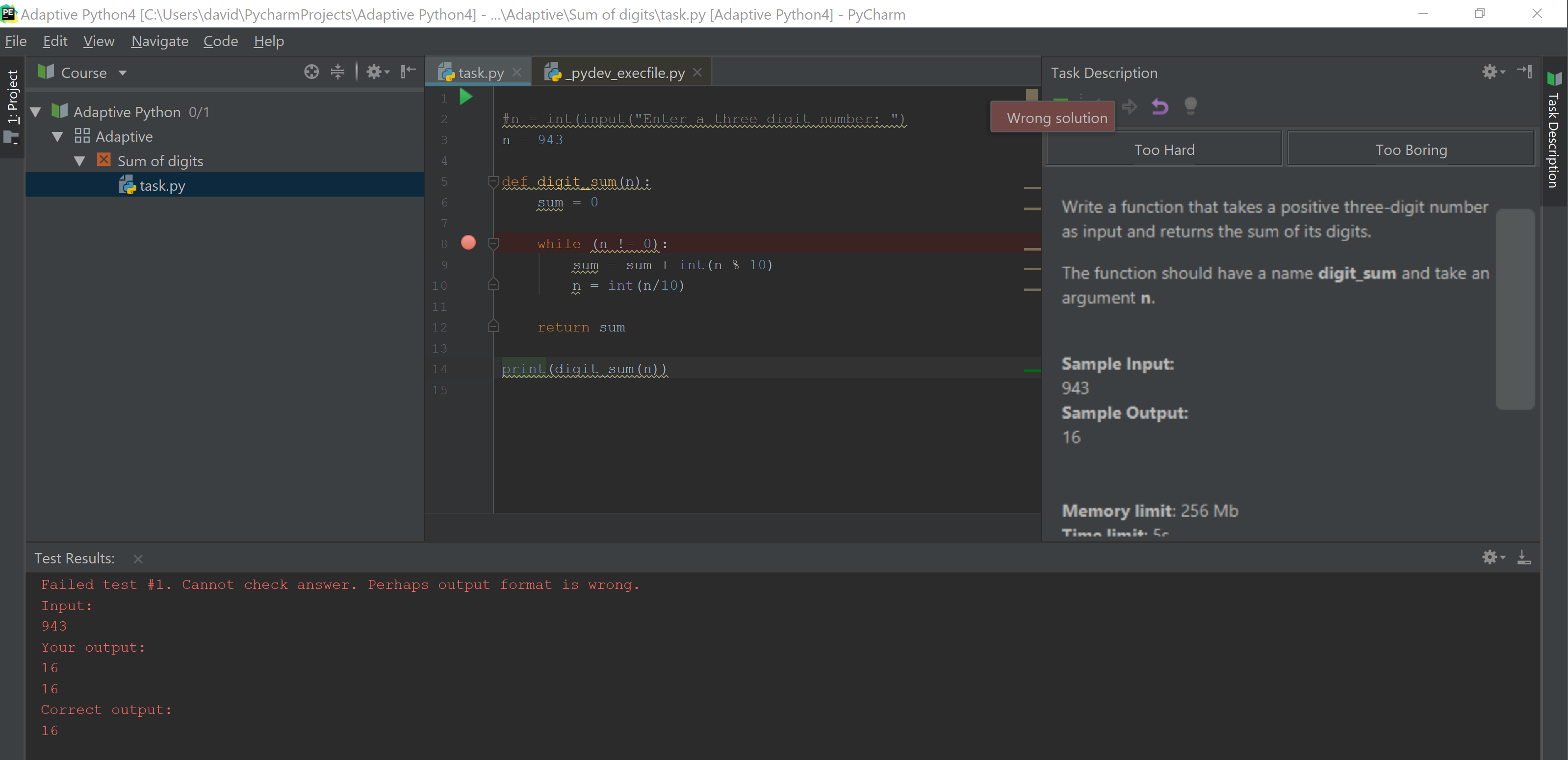So then the absolute final solution and winner goes to…:

```n = 943

def digit_sum(n):
sum = 0

while (n != 0):
sum = sum + int(n % 10)
n = int(n/10)

return sum
```

YES!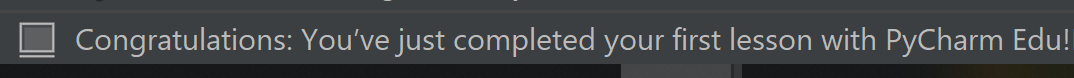## What have I learned from this

So the lesson learned from this is if they ask for a function, then they do not want you to also print the result to the console because they record both the result and the print(value), so to them the answer looks wrong… because you have two results and not just one. Also, my cleverness with the input is also not needed, you just need to give the variable a number, and you are off.

## Conclusion.

Ok so problem number one down, bring on the next 427 others! This by far I have found to be one of the most fun ways to go about practicing the python programming skills as I have to think hard about the problems and how to solve them and when you pass the tasks it is really rewarding, the trick is to never give up, never surrender! The uncomfort you experience from having to get through a task is actually your bodies natural reaction to having to do some actual work; it is like giving your brain a workout much like working out and training any other muscle.

If the estimate is to be believed, I have 101 hours (I love the precision of this) worth of brain workout ahead of me, so in real time this is probably more like 400-500 hours (maybe a million if my math is right).

This is a great way to test yourself and to practice what you know over a range of different kinds of problems. Practice is the key to learning, and this is a good platform to enable it as you can get feedback on your answers and see whether they are right or whether you are well off.
By the end of this, you will have a fully tried and tested skill set and the confidence to take on some hard challenges. So with that said this is the last step in my journey to learning to program Python and the quest to become a developer.

## What now?

Well after I have got through this course, I think I will start on an application of my own. I am by no means a professional programmer, but I think with everything said and done I have the foundations now to be able to at least approach programming and find out how to do any of the things I can’t do already. There are definitely some gaps though, for example, math and equations will probably help a lot as computer programming relies a lot on logic, the code itself is not the hardest part, but knowing what to do with the code to achieve certain things is the incredible part. I also don’t think that this is the kind of skill that you can ever stop learning in, as there is always something new to learn, and different problems to try and solve which makes it kind of exciting and that’s without adding in any of the new technologies and languages that are about.

In terms of projects I especially find myself being drawn to internet technologies and maybe some game development. Which might mean that taking on a different language would be the way to go, for instance, javascript and Kotlin are supposed to be pretty useful in terms of browser-side programming, and C# for game development is apparently a good option. But this is future me’s problem, for now, there is still a lot more I can do with Python.

All fixed set patterns are incapable of adaptability or pliability. The truth is outside of all fixed patterns. – Bruce Lee